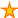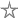# MinOfList() and MaxOfList() functions

22 votes:13,715 views      No comments
by Allen Browne, 20 April 2005    (for Access 97+)

### Microsoft Access: VBA Programming Code

Provided by allenbrowne.com, December 2004. Updated July 2006

# MinOfList() and MaxOfList() functions

Access does not have functions like Min() and Max() in Excel, for selecting the least/greatest value from a list. That makes sense in a relational database, because you store these values in a related table. So Access provides DMin() and DMax() for retrieving the smallest/largest value from the column in the related table.

Occasionally, you still need to pick the minimum or maximum value from a list. The functions below do that. They work with numeric fields, including currency and dates. They return Null if there was no numeric value in the list.

### Using the functions

To create them:

1. Create a new module. In Access 97 - 2003, click the Modules tab of the database window, and click New. In Access 2007, click the Create ribbon, and choose Module (the rightmost icon on the Other group.) Access opens the code window.
2. Copy the code below, and paste into your code window.
3. Check that Access understands the code, by choosing Compile on the Debug menu.
4. Save the module with a name such as Module1.

Use them like any built-in function.

For example, you could put this in a text box:
=MinOfList(5, -3, Null, 0, 2)

Or you could type this into a fresh column of the Field row in a query that has three date fields:
MaxOfList([OrderDate], [InvoiceDate], [DueDate])

```Function MinOfList(ParamArray varValues()) As Variant
Dim i As Integer        'Loop controller.
Dim varMin As Variant   'Smallest value found so far.

varMin = Null           'Initialize to null

For i = LBound(varValues) To UBound(varValues)
If IsNumeric(varValues(i)) Or IsDate(varValues(i)) Then
If varMin <= varValues(i) Then
'do nothing
Else
varMin = varValues(i)
End If
End If
Next

MinOfList = varMin
End Function

Function MaxOfList(ParamArray varValues()) As Variant
Dim i As Integer        'Loop controller.
Dim varMax As Variant   'Largest value found so far.

varMax = Null           'Initialize to null

For i = LBound(varValues) To UBound(varValues)
If IsNumeric(varValues(i)) Or IsDate(varValues(i)) Then
If varMax >= varValues(i) Then
'do nothing
Else
varMax = varValues(i)
End If
End If
Next

MaxOfList = varMax
End Function```

### Understanding the functions

The ParamArray keyword lets you pass in any number of values. The function receives them as an array. You can then examine each value in the array to find the highest or lowest. The LBound() and UBound() functions indicate how many values were passed in, and the loop visits each member in the array.

Any nulls in the list are ignored: they do not pass the IsNumeric() test.

The return value (varMin or VarMax) is initialized to Null, so the function returns Null if no values are found. It also means that if no values have been found yet, the line:
If varMin <= varValues(i) Then
evaluates to Null, and so the Else block executes. Since an If statement has three possible outcomes - True, False, and Null - a "do nothing" for one is a convenient way to handle the other two. If that is new, see Common errors with Null.

Note that the functions would yield wrong results if the return value was not initialized to Null. VBA initializes it to Empty. In numeric comparisons, Empty is treated as zero. Since the function then has a zero already, it would then fail to identify the lowest number in the list.

Rate this article:This is a cached tutorial, reproduced with permission.